﻿ 智能自适应电火花加工

智能自适应电火花加工Intelligent Adaptive Electrical Discharge Machining (EDM)

1. 引言

2. 智能自适应电火花加工控制系统

2.1. 智能自适应电火花系统中的感知功能

“感知”是智能制造的基础，电火花加工的感知功能就是自动识别加工过程中放电间隙内所出现的各种放电脉冲，即开路脉冲、火花放电脉冲、瞬态拉弧脉冲、稳态拉弧脉冲、短路脉冲等。首先需要建立如图1的闭环控制系统，图中D/A代表模拟/数字转换、EDM代表电火花加工、A/D代表数字/模拟转换、IPC代表工控机。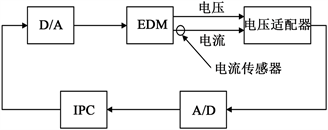Figure 1. A block diagram of closed-loop control system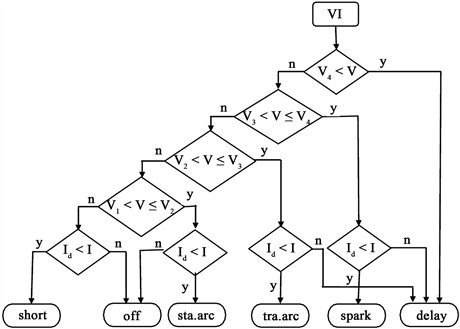Figure 2. A flowchart of identification of discharging pulses

2.2. 智能自适应电火花系统的“认知”功能和“提供最佳行为准则”

2.2.1. 智能自适应电火花系统的“认知”功能

“认知”一：电火花加工放电状态指标－拉弧率

${y}_{1}\left(t\right)=\frac{{\tau }_{stab.arc}+{\tau }_{short}}{{\tau }_{spark}+{\tau }_{tran.arc}+{\tau }_{stab.arc}+{\tau }_{short}+{\tau }_{delay}}$ (1)

“认知”二：反应加工速度的控制指标－有效放电率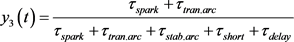(2)

${y}_{3}\left(t\right)$ 为有效放电率。有效放电率越大，加工速度越快；反之，有效放电率越小，加工速度越慢。

${y}_{2}\left(t\right)=\frac{{\tau }_{spark}+{\tau }_{tran.arc}+{\tau }_{stab.arc}+{\tau }_{short}}{{\tau }_{spark}+{\tau }_{tran.arc}+{\tau }_{stab.arc}+{\tau }_{short}+{\tau }_{delay}}$ (3)

${y}_{2}\left(t\right)$ 为放电率。由公式(1)、(2)、(3)可以看出，

${y}_{3}\left(t\right)={y}_{2}\left(t\right)-{y}_{1}\left(t\right)$ (4)

“认知”三：加工环境指标

${y}_{4}\left(t\right)={f}_{corr}\left({y}_{1}\left(t\right),{y}_{1}\left(t-1\right),\cdots ,{y}_{1}\left(t-m\right)\right)$ (5)

2.2.2. “提供未来最佳行为准则”功能

“提供未来最佳行为准则”就是在所确定的加工环境优劣程度的基础上，加工环境所能允许的最佳放电状态期望值。该准则是平衡加工速度与加工稳定性最佳指标，是控制变量控制行为遵守的依据。因此，“最佳行为准则”是达到最优控制的“指导思想”。同时，在当前加工环境下提出的未来加工环境的行为准则，这项功能必须具备对未来环境的预测功能。

2.3. 最优控制行为

$W=U\cdot I\cdot T\left(t\right)\cdot {y}_{3}$ (6)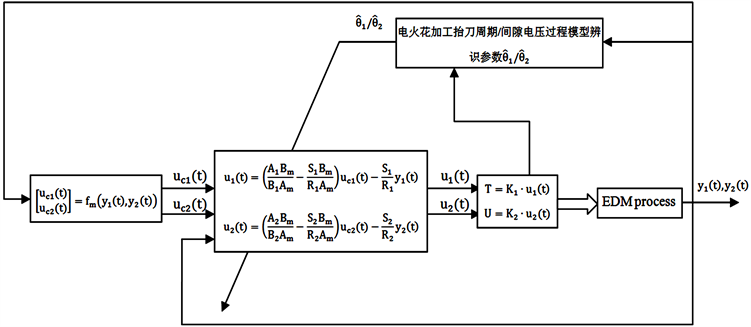Figure 3. A schematic diagram of intelligent adaptive control EDM

2.3.1. 电火花加工环境模型和加工模型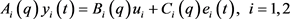(7)

${\stackrel{^}{\theta }}_{ti}=\mathrm{arg}{\mathrm{min}}_{\theta }{\sum }_{k=1}^{t}{\beta }_{i}\left(t,k\right){\left[{y}_{i}\left(k\right)-{\phi }_{i}^{\text{T}}\left(k\right){\theta }_{i}\right]}^{2}$ (8)

${R}_{i}\left(t\right)={\sum }_{k=1}^{t}{\beta }_{i}\left(t,k\right){\phi }_{i}\left(k\right){\phi }_{i}^{\text{T}}\left(k\right)$ (9)

${f}_{i}\left(t\right)={\sum }_{k=1}^{t}{\beta }_{i}\left(t,k\right){\phi }_{i}\left(k\right){y}_{i}\left(k\right)$ (10)

${\stackrel{^}{\theta }}_{ti}\left(t\right)={R}_{i}^{-1}\left(t\right){f}_{i}\left(t\right)$ (11)

${P}_{i}\left(t\right)={R}_{i}^{-1}\left(t\right)$ (12)

$\left\{\begin{array}{l}{\stackrel{^}{\theta }}_{yi}\left(t\right)={\stackrel{^}{\theta }}_{i}\left(t-1\right)+{L}_{i}\left(t\right)\left[{y}_{i}\left(t\right)-{\phi }_{i}^{\text{T}}{\theta }_{i}\left(t-1\right)\right]\\ {L}_{i}\left(t\right)=\frac{{P}_{i}\left(t-1\right){\phi }_{i}\left(t\right)}{{\lambda }_{i}\left(t\right)+{\phi }_{i}^{\text{T}}{P}_{i}\left(t-1\right){\phi }_{i}\left(t\right)}\\ {P}_{i}\left(t\right)=\frac{1}{{\lambda }_{i}\left(t\right)}\left[{P}_{i}\left(t-1\right)-\frac{{P}_{i}\left(t-1\right){\phi }_{i}\left(t\right){\phi }_{i}^{\text{T}}{P}_{i}\left(t-1\right)}{{\lambda }_{i}\lambda \left(t\right)+{\phi }_{i}^{\text{T}}{P}_{i}\left(t-1\right){\phi }_{i}\left(t\right)}\right]\end{array}$ (13)

2.3.2. 控制策略

${R}_{i}{u}_{i}\left(t\right)={T}_{i}{u}_{ci}\left(t\right)-{S}_{i}{y}_{i}\left(t\right),i=1,2$ (14)

${u}_{i}\left(t\right)=\frac{{T}_{i}}{{R}_{i}}{u}_{ci}\left(t\right)-\frac{{S}_{i}}{{R}_{i}}{y}_{i}\left(t\right),i=1,2$ (15)

${y}_{i}\left(t\right)=\frac{{B}_{i}{T}_{i}}{{A}_{i}{R}_{i}+{B}_{i}{S}_{i}}{u}_{ci}\left(t\right)+\frac{{R}_{i}{C}_{i}}{{A}_{i}{R}_{i}+{B}_{i}{S}_{i}}{e}_{i}\left(t\right),i=1,2$ (16)

${A}_{ci}={A}_{i}{R}_{i}+{B}_{i}{S}_{i}$ (17)

$\mathrm{deg}{S}_{i}\le \mathrm{deg}{R}_{i}$

$\mathrm{deg}{T}_{i}\le \mathrm{deg}{R}_{i}$

$\mathrm{deg}{R}_{i}\le \mathrm{deg}{A}_{ci}-\mathrm{deg}{A}_{i}$

$\mathrm{deg}{A}_{ci}\ge 2\mathrm{deg}{A}_{i}-1$

${A}_{ci}={q}^{d-1}{B}_{i}^{+}{C}_{i}={R}_{i}+{B}_{i}{S}_{i}$ (18)

$\frac{{B}_{i}{T}_{i}}{{A}_{i}{R}_{i}+{B}_{i}{S}_{i}}=\frac{{B}_{i}{T}_{i}}{{A}_{ci}}=\frac{{B}_{mi}}{{A}_{mi}},i=1,2$ (19)

${T}_{i}=\frac{{B}_{mi}\left({A}_{i}{R}_{i}+{B}_{i}{S}_{i}\right)}{{B}_{i}{A}_{mi}},i=1,2$ (20)

${u}_{i}\left(t\right)=\left(\frac{{A}_{i}{B}_{mi}}{{B}_{i}{A}_{mi}}+\frac{{S}_{i}{B}_{mi}}{{R}_{i}{A}_{mi}}\right){u}_{ci}\left(t\right)-\frac{{S}_{i}}{{R}_{i}}{y}_{i}\left(t\right),i=1,2$ (21)

3. 实验验证

3.1. 案例一：智能自适应电火花加工钼钛锆(TZM)合金Table 1. The composition of TZM alloy (mass fraction)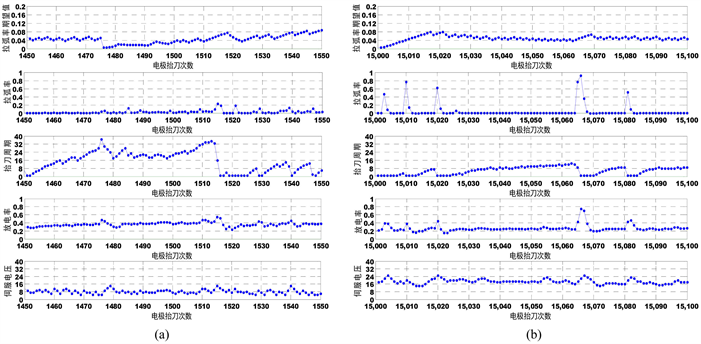Figure 4. The machining stage in good (a); poor (b) machining situation by intelligent adaptive EDM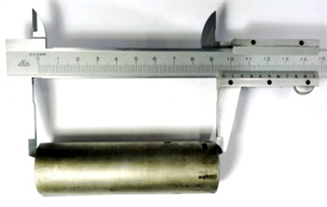Figure 5. The machined hole with molybdenum-titanium-zirconium alloy by intelligent adaptive EDM

3.2. 案例二：智能自适应电火花加工加工Cr12模具钢

Cr12模具钢是高碳高铬型冷作模具钢，含有极高量(质量分数)的C2.00%~2.30%和Cr11.00%~13.00%，属于莱氏体钢，有很高的淬透性、淬硬性和耐磨性，同时也具备抗冲击韧度差，导热性和高温塑性差的特点。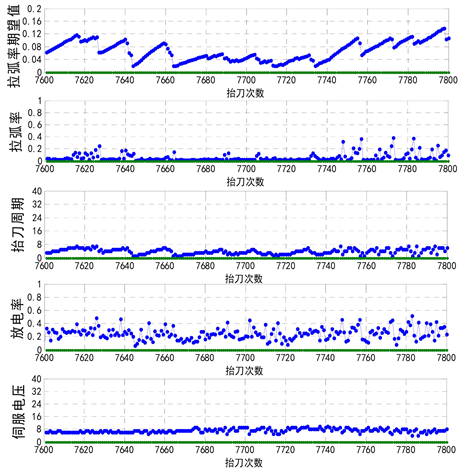(a)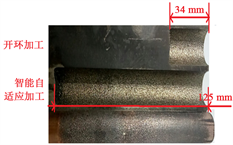(b)

Figure 6. (a) A case of the machining stage in machining Cr12 die steel by intelligent adaptive EDM; (b) a comparison of machined holes with Cr12 by conventional EDM and intelligent adaptive EDM

3.3. 案例三：智能自适应电火花加工加工镍基718合金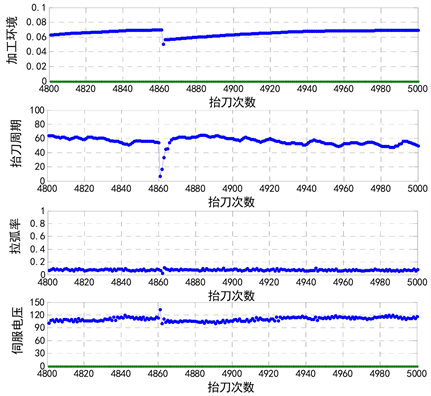(a)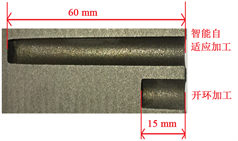(b)

Figure 7. (a) A case of the machining stage in machining Inconel 718 alloy by intelligent adaptive EDM; (b) a comparison of machined holes with Inconel 718 alloy by conventional EDM and intelligent adaptive EDM

4. 结论

 Kunieda, M., Lauwers, B., Rajurkar, K.P., et al. (2005) Advancing EDM through Fundamental Insight into the Process. CIRP Annals, 54, 64-87.
https://doi.org/10.1016/S0007-8506(07)60020-1

 Liu, Y.F. and Pei, P.C. (2006) Asymptotic Analysis on Au-toignition and Explosion Limits of Hydrogen-Oxygen Mixtures in Homogeneous Systems. International Journal of Hydrogen Energy, 31, 639-647.
https://doi.org/10.1016/j.ijhydene.2005.05.005

 Wu, J.Y., Ming, Z., Xu, X.Y., et al. (2015) Fast and Stable Electrical Dis-charge Machining (EDM). Mechanical Systems and Signal Processing, 72, 420-431.

 Zhou, M., Wu, J.Y., Yang, J.W., et al. (2016) Fast and Stable Electrical Discharge Machining (EDM) by Two-Step-Ahead Predicted Control. Procedia CIRP, 42, 215-220.
https://doi.org/10.1016/j.procir.2016.02.274

 Zhou, M., Han, F., Wang, Y., et al. (2009) Assessment of the Dynamical Properties in EDM Process-Detecting Deterministic Nonlinearity of EDM Process. International Journal of Advanced Manufacturing Technology, 44, 91-99.
https://doi.org/10.1007/s00170-008-1817-6

 Zhou, M., Meng, X.Y., Qin, J.J., Chen, Z.G. and Lian, X.J. (2013) Building an EDM Process Model by an Instrumental Variable Approach Based on Two Interactive Kalman Filters. Precision Engineering, 37, 146-158.
https://doi.org/10.1016/j.precisioneng.2012.07.011

 Zhou, M., Han, F.Z. and Soichiro, I. (2008) A Time-Varied Predictive Model for EDM Process. International Journal of Machine Tools and Manufacture, 48, 1668-1677.
https://doi.org/10.1016/j.ijmachtools.2008.07.003

 Ljung, L. (1999) System Identification—Theory for the User. 2nd Edition, Prentice-Hall PTR, 363.

 Zhou, M., Wu, J.Y., Xu, X.Y., Mu, X. and Dou, Y.P. (2018) Significant Improvements of Electrical Discharge Machining Performance by Step-by-Step Updated Adaptive Control Laws. Mechanical Systems and Signal Processing, 101, 480-497.
https://doi.org/10.1016/j.ymssp.2017.06.041

 王东辉, 袁晓波, 李中奎, 等. 钼及钼合金研究与应用进展[J]. 中国材料进展, 2006, 25(12): 1-7.

 黄金昌. 钼和钼合金及其加工新动向[J]. 中国钼业, 1994(6): 22-25.

 钟培全. 钼与钼合金的应用及其加工方法[J]. 中国钼业, 2000, 24(5): 15-16.

 吴新光, 杜晓斌. TZM合金及其特性[J]. 中国钼业, 2005, 29(5): 30-31.

 杨宇锋. 钼和钼合金深加工技术进展[J]. 中国钼业, 2001, 25(4): 34-37.

 孙长宏, 朱颖谋, 王佳兴, 等. 钼钛锆合金材料特殊型腔的组合放电加工方法的研究[J]. 导航与控制, 2016, 15(4).

 朱颖谋, 高飞, 孙长宏, 等. 钼钛锆高温合金的电火花加工工艺研究[J]. 航空制造技术, 2016, 509(14): 61-64.

 朱颖谋, 孙长宏, 牛禄, 等. 钼钛锆高温合金电火花线切割加工效率优化[J]. 电加工与模具, 2015(5): 8-12.

Top